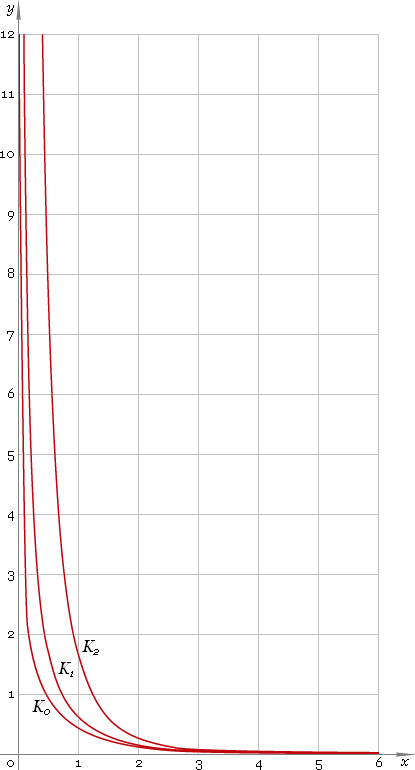The Art of Interface

# K — modified Bessel function of the second kind

Category. Mathematics.

Abstract. Modified Bessel function of the second kind of the real (fractional) order: definition, plot, properties and identities.

## 1. Definition

By definition modified Bessel function is solution of the modified Bessel equation

z2 w′′ + z w′ − (z2 + ν2) w = 0

As second order equation it has two solutions, second of which has singularity at 0 and is called modified Bessel function of the second kind — Kν. Parameter ν is called order of the function.

First solution has no singularity at 0 and is called modified Bessel function of the first kind — Iν.

## 2. Plot

Modified Bessel functions of the second kind defined everywhere on the real axis, at 0 functions have singularity, so, their domain is (−∞, 0)∪(0, +∞). Plots of the first three representatives of the second kind modified Bessel function family depicted below — fig. 1.Fig. 1. Plots of the modified Bessel functions of the second kind y = K0(x), y = K1(x) and y = K2(x).

## 3. Identities

Connection to the ordinary Bessel functions:

Kν(z) = π /2 iν+1[Jν(iz) + iYν(iz)]

Connection to the modified Bessel function of the first kind:

Kν(z) = π / [2 sin(πν)] [I−ν(z) − Iν(z)]

Next order recurrence:

Kν+1(z) = 2ν /z Kν(z) + Kν−1(z)

Negative argument:

Kν(−z) = eiπν Kν(z) − i π Iν(z) = cos(πν) Kν(z) − i [sin(πν) Kν(z) + π Iν(z)]

For the case of integer order ν=n the negative argument identity can be simplified down to:

Kn(−z) = (−1)n Kn(z) − i π In(z)

and for the case of half-integer order ν=n+1/2 the identity can be simplified down to:

Kn+1/2(−z) = −i [(−1)n Kn+1/2(z) + π In+1/2(z)]

Reflection — negative order:

K−ν(z) = Kν(z)

## 4. Support

Modified Bessel function of the second kind Kν of the real (fractional) order and complex argument is supported by professional version of the Librow calculator.

## 5. Interface

Modified Bessel function call looks like

``K(order, argument);``

or

``BesselK(order, argument);``

where order is the function real order, and argument — function argument.

## 6. How to use

To calculate modified Bessel function of the second kind of the 0 order of the number:

``K(0, 1.5−i);``

or:

``BesselK(0, 1.5−i);``

To calculate modified Bessel function of the second kind of the 1.2 order of the current result:

``K(1.2, rslt);``

or:

``BesselK(1.2, rslt);``

To calculate modified Bessel function of the second kind of the 2.5 order of the number z in memory:

``K(2.5, mem[z]);``

or:

``BesselK(2.5, mem[z]);``